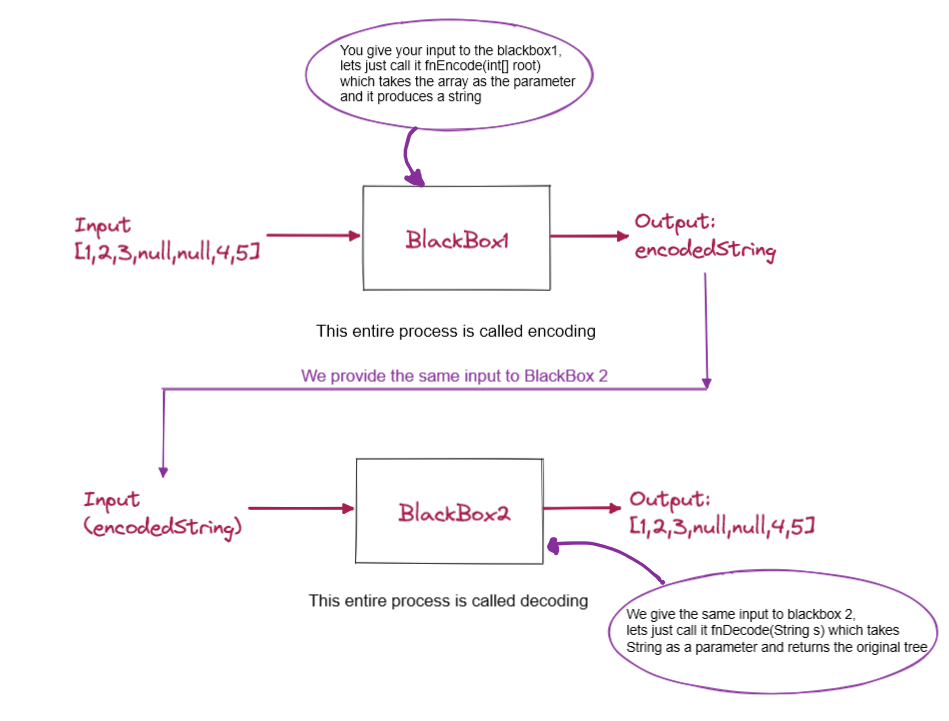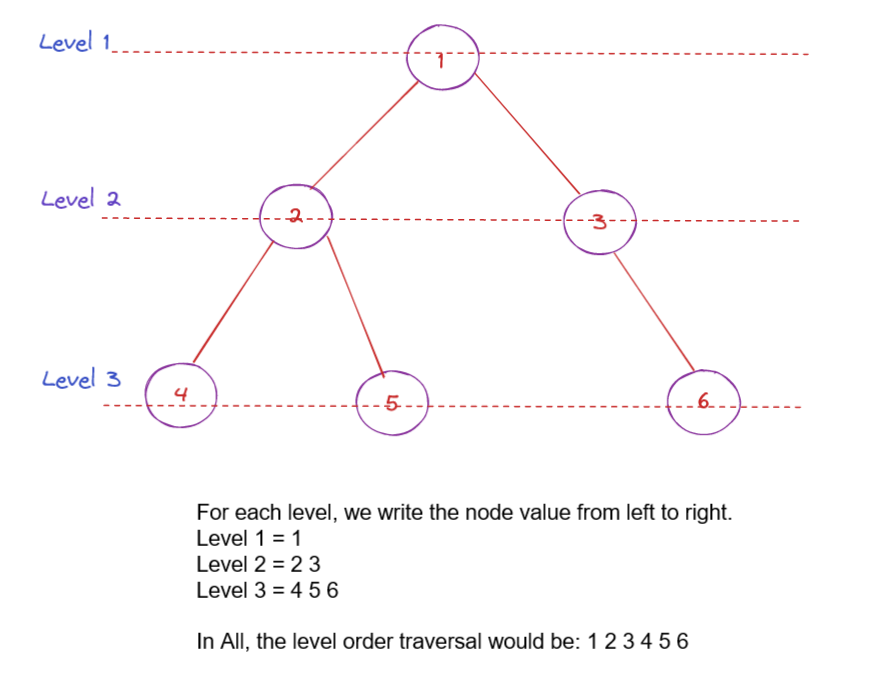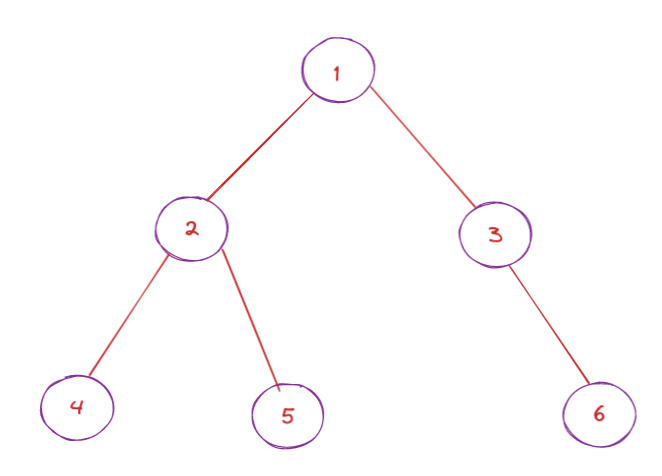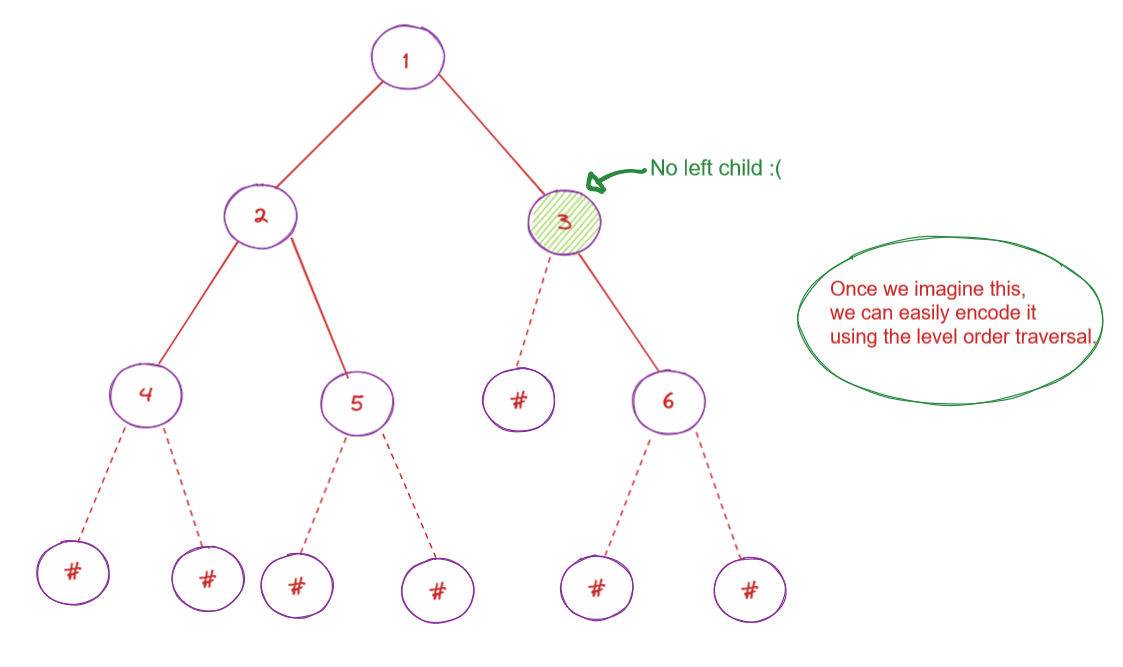# Serialize And Deserialize a Binary Tree

Problem Statement: Design an algorithm to serialize and deserialize a binary tree. There is no restriction on how your serialization/deserialization algorithm should work. You just need to ensure that a binary tree can be serialized to a string and this string can be deserialized to the original tree structure.

Examples:

```Example 1:

Input: root = [1,2,3,null,null,4,5]

Output: [1,2,3,null,null,4,5]

Explanation: The input and the output would be the same for this problem as we are serializing the tree and again deserializing the string format to the binary tree format.

Example 2:

Input:  root = []

Result: []
```

### Solution:

Disclaimer: Don’t jump directly to the solution, try it out yourself first.

Intuition:Approach:

Now let’s dive into the different approaches we have.

Now, there are a lot of ways to solve this particular problem like inorder traversal, preorder traversal, post-order traversal.

We will be solving this problem using the Level Order Traversal. Now just to give a basic gist, what happens in level order traversal is that we go through each level and print the node value.The above diagram pretty much sums up the level order traversal of the binary tree.

Now, let’s get back to the encoding and decoding problem.

Using the level order traversal, we are going to encode the given binary tree. Let’s see how.

Let’s consider the following tree:Notice that node 3 does not have a left child and there is no way to identify that 3’s left child is missing. To solve this problem, we substitute node 3’s left child with some value to indicate that the left child is missing.

So we can substitute with any character except for a number since the node values are numbers already, we would get confused.

The character that we are going to use to substitute is “#”.

Now substitution doesn’t mean that we are going to modify the input data, it is just for our understanding to solve this particular problem. We will understand how exactly we are going to add the value in the code.

Now, let’s imagine the binary tree with our substitution method. The tree would now look like this:Now we do a simple level order traversal and we achieve the following result “1,2,3,4,5,#,6,#,#,#,#,#,#”

Let’s now learn how to deserialize the string:

This is the encoded string: “12345#6######”. So we start from the left and we will use the queue data structure to solve this BlackBox 2.

So, we take the first guy that is 1 and insert it into the queue. Make sure, if the input string is empty or null, it means that there is no tree.

Alright, so 1 is inserted into the queue and we create a root node with the value as 1.

Now, we start iterating on the queue and we say 1s left would be the next character present in the string. In our case it is 2, so we put that in the queue and also assign it as the left child of 1.

Now the right child of 1 would be 3 in our case, that is the next character in the string. We assign 3 as the right child of 1 and put it into the queue. Now 1 is done.

We take the next element from the queue and repeat the same process. Whenever we encounter a # we know that the value of that particular node is null, so we assign null and we won’t be putting that into the queue.

In the end, we would have our decoded Binary Tree.

Code:

## C++ Code

``````class Codec {
public:

// Encodes a tree to a single string.
string serialize(TreeNode* root) {
if(!root) return "";

string s ="";
queue<TreeNode*> q;
q.push(root);
while(!q.empty()) {
TreeNode* curNode = q.front();
q.pop();
if(curNode==NULL) s.append("#,");
else s.append(to_string(curNode->val)+',');
if(curNode != NULL){
q.push(curNode->left);
q.push(curNode->right);
}
}
return s;
}

// Decodes your encoded data to tree.
TreeNode* deserialize(string data) {
if(data.size() == 0) return NULL;
stringstream s(data);
string str;
getline(s, str, ',');
TreeNode *root = new TreeNode(stoi(str));
queue<TreeNode*> q;
q.push(root);
while(!q.empty()) {

TreeNode *node = q.front();
q.pop();

getline(s, str, ',');
if(str == "#") {
node->left = NULL;
}
else {
TreeNode* leftNode = new TreeNode(stoi(str));
node->left = leftNode;
q.push(leftNode);
}

getline(s, str, ',');
if(str == "#") {
node->right = NULL;
}
else {
TreeNode* rightNode = new TreeNode(stoi(str));
node->right = rightNode;
q.push(rightNode);
}
}
return root;
}
};``````

Time Complexity: O(N)

Space Complexity: O(N)

## Java Code

``````public class Codec {
public String serialize(TreeNode root) {
if (root == null) return "";
StringBuilder res = new StringBuilder();
while (!q.isEmpty()) {
TreeNode node = q.poll();
if (node == null) {
res.append("n ");
continue;
}
res.append(node.val + " ");
}
return res.toString();
}

public TreeNode deserialize(String data) {
if (data == "") return null;
String[] values = data.split(" ");
TreeNode root = new TreeNode(Integer.parseInt(values));
for (int i = 1; i < values.length; i++) {
TreeNode parent = q.poll();
if (!values[i].equals("n")) {
TreeNode left = new TreeNode(Integer.parseInt(values[i]));
parent.left = left;
}
if (!values[++i].equals("n")) {
TreeNode right = new TreeNode(Integer.parseInt(values[i]));
parent.right = right;